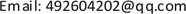1广州供电局电力试验研究院，广东 广州

2西安交通大学电气工程学院，陕西 西安1. 引言

2. 云模型的基本概念

3. 二维分段云模型

3.1. 时间序列的分段策略

L = ∑ i = 1 w l e n g t h ( S i ) = N + ( W − 1 ) × M

O = ( W − 1 ) × M / L × 100 %

S i = ( t i × ( Q + 1 − M ) + 1 , t i × ( Q + 1 − M ) + 2 , ⋯ , t i × ( Q + 1 − M ) + Q )

S W − j + i = ( t N − ( j − 1 ) × ( Q − M ) − Q + 1 , t N − ( j − 1 ) × ( Q − M ) − Q + 2 , ⋯ , t N − ( j − 1 ) × ( Q − M ) )

3.2. 二维分段云模型间的相似性度量

T = ( t 1 , t 2 , ⋯ , t n ) , T ¯ = ( t ¯ 1 , t ¯ 2 , ⋯ , t ¯ n )

T ′ = ( t ′ 1 , t ′ 2 , ⋯ , t ′ w ) , T ¯ ′ = ( t ¯ ′ 1 , t ¯ ′ 2 , ⋯ , t ¯ ′ w )

t ′ i = ( T 1 , Δ T 1 ) = ( ( t 1 , e 1 ) , ⋯ , ( t d 1 , e d 1 ) ) ,         t ¯ ′ i = ( T ¯ 1 , Δ T ¯ 1 ) = ( ( t ¯ 1 , e ¯ 1 ) , ⋯ , ( t ¯ d 1 , e ¯ d 1 ) )

E x = m e a n ( X ) = 1 n ∑ i = 1 n x i

E y = m e a n ( Y ) = 1 n ∑ i = 1 n y i

E n x = 1 n π 2 ∑ i = 1 n | x i − E x |

E n y = 1 n π 2 ∑ i = 1 n | y i − E y |

H e x = v a r ( X ) − E n x 2

{ C 1 ( E x 1 , E y 1 , E n x 1 , E n y 1 , H e x 1 , H e y 1 ) C 2 ( E x 2 , E y 2 , E n x 2 , E n y 2 , H e x 2 , H e y 2 )                                                 ⋮ C w ( E x w , E y w , E n x w , E n y w , H e x w , H e y w ) { C ¯ 1 ( E ¯ x 1 , E ¯ y 1 , E ¯ n x 1 , E ¯ n y 1 , H ¯ e x 1 , H ¯ e y 1 ) C ¯ 2 ( E ¯ x 2 , E ¯ y 2 , E ¯ n x 2 , E ¯ n y 2 , H ¯ e x 2 , H ¯ e y 2 )                                                 ⋮ C ¯ w ( E ¯ x w , E ¯ y w , E ¯ n x w , E ¯ n y w , H ¯ e x w , H ¯ e y w )

S M ( T ′ , T ¯ ′ ) = 1 w ∑ i = 1 w T C M i

4. 实验结果与分析

t i-nm = t i − t min t max − t min

t u = ∑ i = 1 m t i

t σ = 1 m ∑ i = 1 m ( t i − t μ ) 2

t i-sd = t i − t u t σ

Comparison of the experimental results of the two model

DTW79.2%55.9%51.2%62.1%

5. 结论